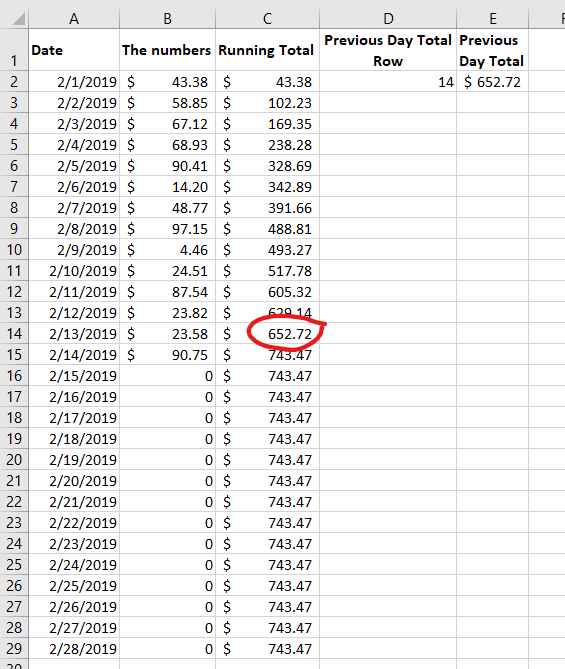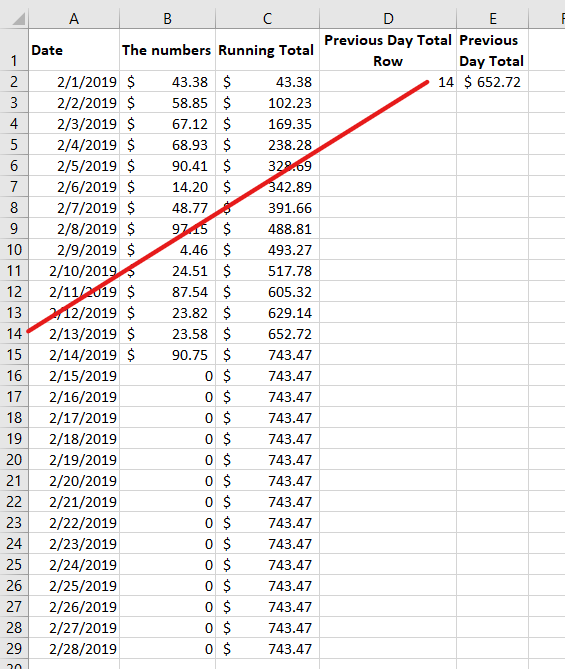# Excel – Previous Day Total

Here is a scenario: A running total of numbers, updated daily. You want to capture the previous day’s total, as you can see in the picture below.Looking for the Previous Day’s total in a column of numbers

I’m showing the answer in two steps here, in real life I’d make it into one formula.
The first step is to capture the row number of the previous day’s total. Finding it using the numbers in Column C would be way too complicated. But Column B has the kind of data we can use.
Using the formula `=LOOKUP(2,1/(B2:B29<>0),ROW(B:B))` captures the row.Finding the row number for the previous day’s value.

What the lookup formula is doing is starting by evaluating the numbers from B2:B29 looking for values that aren’t equal to zero.
This creates an array like this: {TRUE;TRUE;TRUE;TRUE;TRUE;TRUE;TRUE;TRUE;TRUE;TRUE;TRUE;TRUE;TRUE;TRUE;FALSE;FALSE;FALSE;FALSE;FALSE;FALSE;FALSE;FALSE;FALSE;FALSE;FALSE;FALSE;FALSE;FALSE}
The TRUE values will equal 1 and the FALSE values equal 0.
This means when the formula divides 1 by those values, an array looking like this is created:
{1;1;1;1;1;1;1;1;1;1;1;1;1;1;#DIV/0!;#DIV/0!;#DIV/0!;#DIV/0!;#DIV/0!;#DIV/0!;#DIV/0!;#DIV/0!;#DIV/0!;#DIV/0!;#DIV/0!;#DIV/0!;#DIV/0!;#DIV/0!}
Lookup can’t find 2 in the array, so it settles for the largest value in the array that is less than or equal to lookup_value.The ROW function tells it to return the row number from the array B2:B29.
The second step is to combine the row number with the column number and show the result using `=INDIRECT("C"&D2)`
And there you have it. A quick way of always finding the previous day (month, year, whatever).

This site uses Akismet to reduce spam. Learn how your comment data is processed.